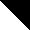# MINLPLib

### A Library of Mixed-Integer and Continuous Nonlinear Programming Instances

#### Instance cvxnonsep_nsig30

```convex MINLP test problem with non-separable signomial constraint function
 Formatsⓘ ams gms mod nl osil py Primal Bounds (infeas ≤ 1e-08)ⓘ 133.31874180 p1 ( gdx sol ) (infeas: 1e-16)131.61545530 p2 ( gdx sol ) (infeas: 0)130.63345720 p3 ( gdx sol ) (infeas: 0)130.62871260 p4 ( gdx sol ) (infeas: 1e-10) Other points (infeas > 1e-08)ⓘ Dual Boundsⓘ 125.76693430 (ALPHAECP)16.86054456 (ANTIGONE)130.62871250 (BARON)130.62871260 (BONMIN)130.62871260 (LINDO)126.08023180 (SCIP)130.62821640 (SHOT) Referencesⓘ Kronqvist, Jan, Lundell, Andreas, and Westerlund, Tapio, Reformulations for utilizing separability when solving convex MINLP problems, submitted, 2017. Applicationⓘ Test Problem Added to libraryⓘ 11 Sep 2017 Problem typeⓘ MINLP #Variablesⓘ 30 #Binary Variablesⓘ 0 #Integer Variablesⓘ 15 #Nonlinear Variablesⓘ 30 #Nonlinear Binary Variablesⓘ 0 #Nonlinear Integer Variablesⓘ 15 Objective Senseⓘ min Objective typeⓘ linear Objective curvatureⓘ linear #Nonzeros in Objectiveⓘ 30 #Nonlinear Nonzeros in Objectiveⓘ 0 #Constraintsⓘ 1 #Linear Constraintsⓘ 0 #Quadratic Constraintsⓘ 0 #Polynomial Constraintsⓘ 0 #Signomial Constraintsⓘ 1 #General Nonlinear Constraintsⓘ 0 Operands in Gen. Nonlin. Functionsⓘ Constraints curvatureⓘ convex #Nonzeros in Jacobianⓘ 30 #Nonlinear Nonzeros in Jacobianⓘ 30 #Nonzeros in (Upper-Left) Hessian of Lagrangianⓘ 900 #Nonzeros in Diagonal of Hessian of Lagrangianⓘ 30 #Blocks in Hessian of Lagrangianⓘ 1 Minimal blocksize in Hessian of Lagrangianⓘ 30 Maximal blocksize in Hessian of Lagrangianⓘ 30 Average blocksize in Hessian of Lagrangianⓘ 30.0 #Semicontinuitiesⓘ 0 #Nonlinear Semicontinuitiesⓘ 0 #SOS type 1ⓘ 0 #SOS type 2ⓘ 0 Minimal coefficientⓘ 4.0000e-03 Maximal coefficientⓘ 1.8800e+00 Infeasibility of initial pointⓘ 0.9989 Sparsity JacobianⓘSparsity Hessian of Lagrangianⓘ```\$offlisting
*
*  Equation counts
*      Total        E        G        L        N        X        C        B
*          2        1        0        1        0        0        0        0
*
*  Variable counts
*                   x        b        i      s1s      s2s       sc       si
*      Total     cont   binary  integer     sos1     sos2    scont     sint
*         31       16        0       15        0        0        0        0
*  FX      0
*
*  Nonzero counts
*      Total    const       NL      DLL
*         61       31       30        0
*
*  Solve m using MINLP minimizing objvar;

Variables  i1,i2,i3,i4,i5,i6,i7,i8,i9,i10,i11,i12,i13,i14,i15,x16,x17,x18,x19
,x20,x21,x22,x23,x24,x25,x26,x27,x28,x29,x30,objvar;

Integer Variables  i1,i2,i3,i4,i5,i6,i7,i8,i9,i10,i11,i12,i13,i14,i15;

Equations  e1,e2;

e1..    1.29*i1 + 0.76*i2 + i3 + 1.62*i4 + 1.07*i5 + 0.7*i6 + 1.88*i7 + 1.75*i8
+ 1.1*i9 + 1.24*i10 + 1.17*i11 + 0.82*i12 + 1.06*i13 + 0.94*i14
+ 0.46*i15 + 1.69*x16 + 0.39*x17 + 0.45*x18 + 0.34*x19 + 0.46*x20
+ 1.86*x21 + 1.46*x22 + 0.98*x23 + 1.16*x24 + 0.47*x25 + 0.92*x26
+ 1.43*x27 + 1.81*x28 + 1.78*x29 + 0.67*x30 - objvar =E= 0;

e2.. -0.2*i1**0.028*i2**0.041*i3**0.047*i4**0.022*i5**0.041*i6**0.026*i7**0.052
*i8**0.051*i9**0.016*i10**0.038*i11**0.036*i12**0.033*i13**0.054*i14**
0.016*i15**0.02*x16**0.007*x17**0.058*x18**0.04*x19**0.03*x20**0.039*x21**
0.034*x22**0.04*x23**0.034*x24**0.044*x25**0.032*x26**0.061*x27**0.013*x28
**0.007*x29**0.007*x30**0.004 =L= -1;

* set non-default bounds
i1.lo = 1; i1.up = 10;
i2.lo = 1; i2.up = 10;
i3.lo = 1; i3.up = 10;
i4.lo = 1; i4.up = 10;
i5.lo = 1; i5.up = 10;
i6.lo = 1; i6.up = 10;
i7.lo = 1; i7.up = 10;
i8.lo = 1; i8.up = 10;
i9.lo = 1; i9.up = 10;
i10.lo = 1; i10.up = 10;
i11.lo = 1; i11.up = 10;
i12.lo = 1; i12.up = 10;
i13.lo = 1; i13.up = 10;
i14.lo = 1; i14.up = 10;
i15.lo = 1; i15.up = 10;
x16.lo = 1E-5; x16.up = 10;
x17.lo = 1E-5; x17.up = 10;
x18.lo = 1E-5; x18.up = 10;
x19.lo = 1E-5; x19.up = 10;
x20.lo = 1E-5; x20.up = 10;
x21.lo = 1E-5; x21.up = 10;
x22.lo = 1E-5; x22.up = 10;
x23.lo = 1E-5; x23.up = 10;
x24.lo = 1E-5; x24.up = 10;
x25.lo = 1E-5; x25.up = 10;
x26.lo = 1E-5; x26.up = 10;
x27.lo = 1E-5; x27.up = 10;
x28.lo = 1E-5; x28.up = 10;
x29.lo = 1E-5; x29.up = 10;
x30.lo = 1E-5; x30.up = 10;

Model m / all /;

m.limrow=0; m.limcol=0;
m.tolproj=0.0;

\$if NOT '%gams.u1%' == '' \$include '%gams.u1%'

\$if not set MINLP \$set MINLP MINLP
Solve m using %MINLP% minimizing objvar;

```

Last updated: 2023-08-16 Git hash: 2519540e